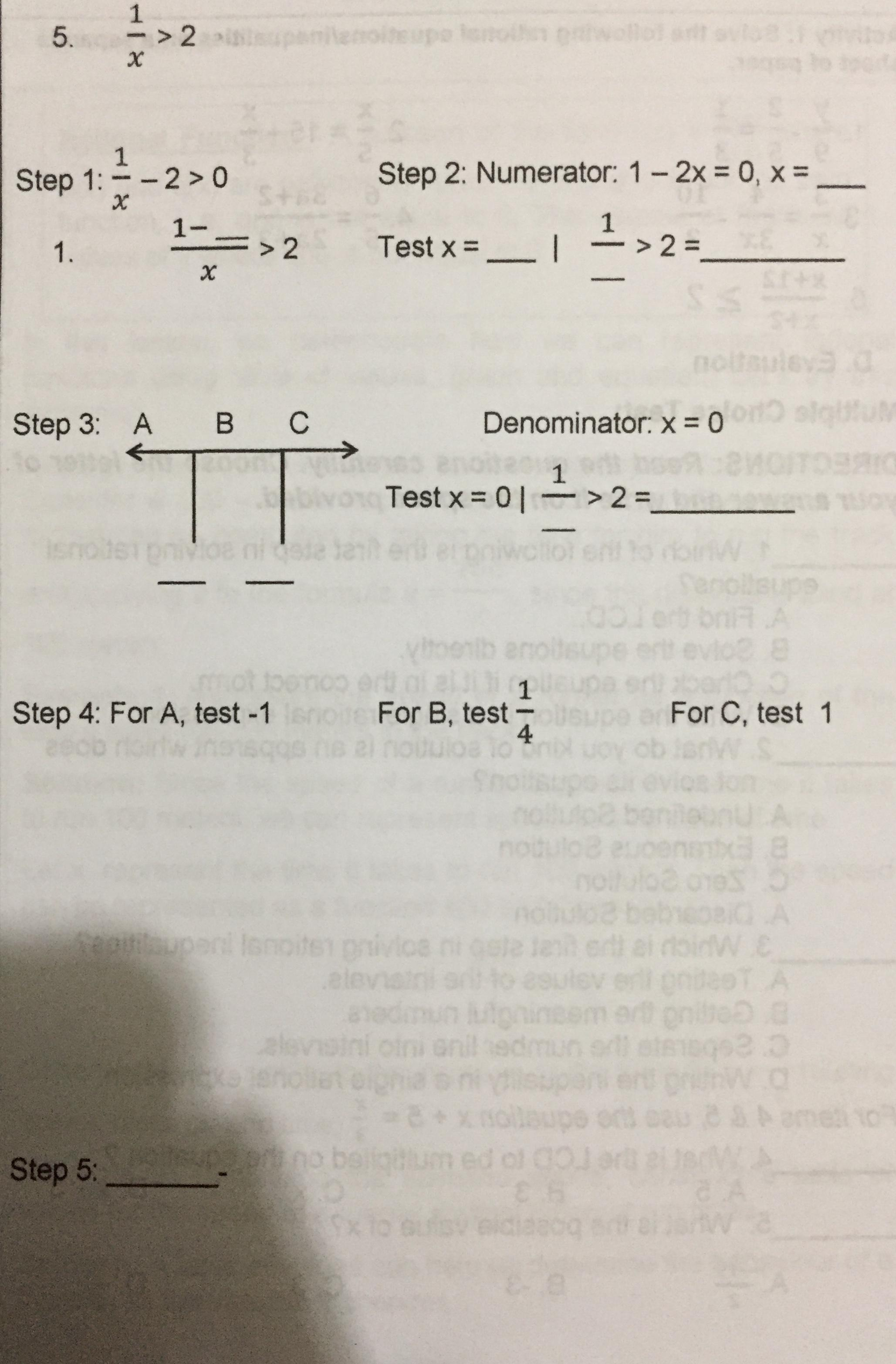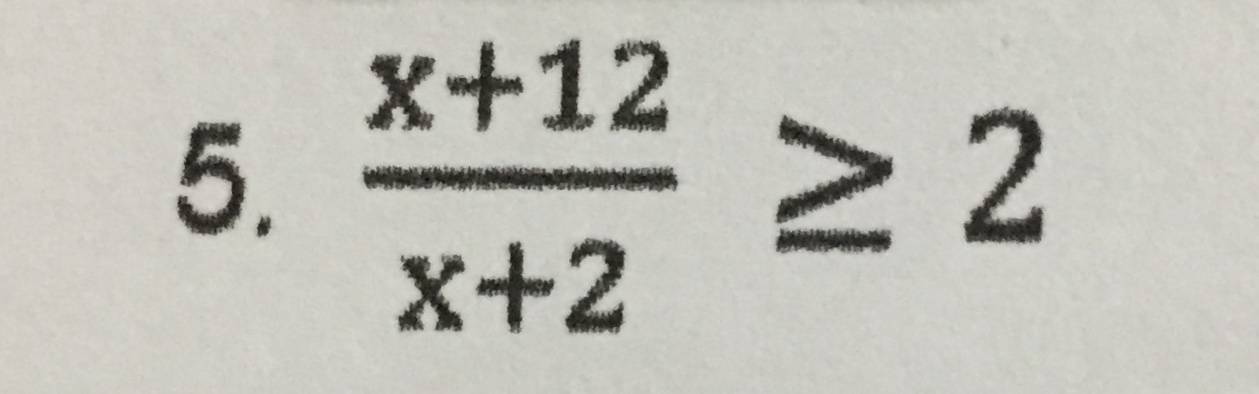# Rational Inequalities

• MHB
Achi_kunskeeter
Uhh ... fill in the blanks? Have you worked on any of these?

Achi_kun
Uhh ... fill in the blanks? Have you worked on any of these?
Idk how the solution works

skeeter
for $\dfrac{1}{x} > 2$

step 1. $\dfrac{1}{x} - \dfrac{2}{1} > 0$

combine the two fractions by using a common denominator

HOI
Personally, I wouldn't do it that say.
From $\frac{1}{x}> 2%$, multiply on both sides by x.
But you have to be careful with that! Unlike with an equation, multiplying on both sides by negative number reverses the ">" sign. So do two cases:

1) If x> 0 then $1>2x$. Divide on both sides by the positive number 2: $\frac{1}{2}> x$..
Since we are requiring that x be positve, we have $0< x< \frac{1}{2}$

2) If x< 0 then $1< 2x$. Divide on both sides by the positive number 2: $\frac{1}{2}< x$, But since we are requiring that x be negative, that is not possible.

The solution is $0<x \frac{1}{2}$.

It is also true that, for continuous functions, g and f, to change from f(x)<g(x) to f(x)> g(x), we have to go through f(x)= g(x) or a poinr where either f or g is undefined.

So start by solving the equation $\frac{1}{x}= 2$. That is the same as $1= 2x$, or $x=\frac{1}{2}$. I is also true that $\frac{1}{x}$ is undefined for x= 0. That divides the real numbers into three intervals, x< 0, 0< x< 1/2, and x> 1/2. We need only check one value of x in each interval. For x< 0 take x=-1. Then 1/x= -1 which is NOT larger than 2 so no x less than 0 satisfies 1/x> 2. For 0< x< 1/2 we can take x=1/4. Then 1/x= 4 which is greater than 2. Every number betwen 0 and 1/2 satisfies the inequalty. Finally take x= 1. Then 1/x=1 which is not larger than 2. No x larger than 1/2 satisfies the inequality.